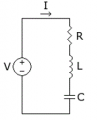# Series RLC circuit connected to AC voltage generator.

#### cdummie

Joined Feb 6, 2015
124
So i have RLC circuit where R, L and C parameters are known, this circuit is connected to ideal AC voltage generator with effective value of voltage E. Find all the values of angular frequency for which effective value of current in the circuit is equal to Imax/sqrt(2) where Imax=E/R is maximal effective value of current in the moments when this circuit is in resonant frequency.Now, when it comes to resonant frequency is relatively simple, since that is the frequency when impedance is lowest possible. Impedance here is Z=R+ j(ωL - 1/(ωC)) it means that lowest value of impedance will be when ωL=1/(ωC) or
ω=1/sqrt(LC) or f=1/(2πsqrt(LC)) and that's what's going on with frequency when we have maximal value of current, but how can i find frequencies (i believe there should be two frequencies where I=Imax/sqrt(2)) such that they satisfy the requirement of this example.

#### Jony130

Joined Feb 17, 2009
5,445
i believe there should be two frequencies where I=Imax/sqrt(2)
Can you explain why two (real) frequency ??